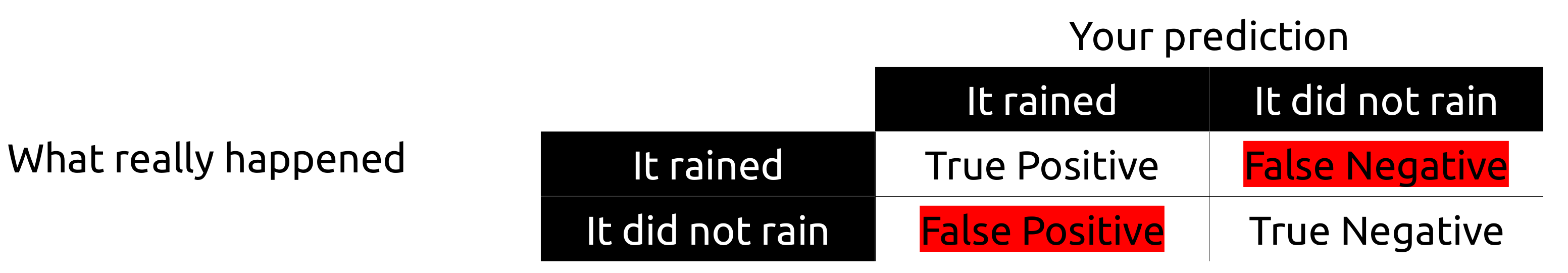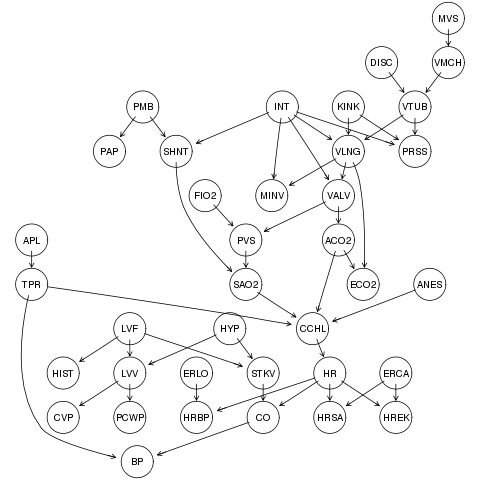# How can I evaluate my model? Part I.

One way to evaluate your model is in terms of error types. Let’s consider a scenario where you live in a city where it rains every once in a while. If you guessed that it would rain this morning, but it did not, your guess was a false positive, sometimes abbreviated as FP. If you said it would not rain, but it did, then you had a false negative (FN). Raining when you do not have an umbrella may be annoying, but life is not always that bad. You could have predicted that it would rain and it did (true positive, TP) or predicted that it would not rain and it did not (true negative, TN). In this example, it’s easy to see that in some contexts one error may be worse than the other and this will vary according to the problem. Bringing an umbrella with you in a day with no rain is not as bad as not bringing an umbrella on a rainy day, right?

It doesn’t really make sense to say that a true negative or a true positive is an error. That’s why we only have two error types. Error Type I occurs when you predicted that an event would occur but it did not (false positive, FP) and Error Type II occurs when you predicted that one event would not occur but it did occur (false negative, FN).Possible outcomes organized as a true positive, a false negative, a false positive and a true negative outcome. Error types I and II are highlighted in red.

It may be that one of your goals, when you are creating a model, is to lower the error type I or error type II, depending on what you’re trying to do. If this is the case, you will probably analyze your predictions in terms of recall, precision and other equations that make use of these two. Before telling you what recall and precision are, I will show you how they’re defined (equation images generated with codecogs equation editor):

Since I work with network discovery, and I think it’s easier to teach this by thinking on networks, from now on the examples will be illustrated by networks. The basic terminology in graph theory, having in mind what will be discussed here, is comprised of: nodes (represented here with a circle and an identifier inside it, meaning a property of your model) and edges (lines that represent an association of some kind between two nodes). One example of a network can be seen below:The alarm dataset (A Logical Alarm Reduction Mechanism) is designed to provide an alarm message system for patient monitoring and contains 37 variables. The network image and meta information were retrieved from here and here.

## Recall

Recall, also known as sensitivity, is the measure of how many positive results (TP) your model was able to recover, not worrying about false positives (FP). Here, it’s better to bring an edge (even if it does not exist) than to not bring one a tall. If you have correctly inferred one edge, as long as you do not make a false negative mistake (saying there is no edge where in reality there is one) you will obtain the maximum recall, which is 1. As you can see here, recall is penalized by false negative results. If error type II is worse than error type I for your problem, recall may be what you’re looking for.

## Precision

Precision, also known as the positive predictive value (PPV), is the measure of how many positive results (TP) your model was able to recover, with an effort to not bring false positives. Here, it’s better to not bring an edge (even if it exists) than to bring an edge at all. If you have correctly inferred one edge, as long as you do not have any false positive mistake (saying there is an edge where in reality there is none) you will obtain the maximum precision, which is 1. As you can also see here, precision is penalized by false positive results. If error type I is worse than error type II for your problem, precision may be what you’re looking for.

You may have noticed that in both descriptions I mentioned the same idea: as long as you have inferred at least one correct edge. If this is not clear for you, have a look at the definitions of recall and precision again. There, you have the true positive, TP, in both the numerator and denominator. So if FN or FP is 0, as long as TP=1 you have 1/1 = 1, either for recall or precision.

If you do not have any true positive and you did not draw an edge that should be there (FN=1, for example), then you have:

If you do not have any true positive and you drew an edge that should not be there (FP=1, for example), then you have:

## Specificity

Specificity is also known as the true negative rate (TNR). It measures the proportion of actual negatives that are correctly identified as such (e.g., the percentage of edges that are missing and indeed should not be in our model). If the last thing you want is to not bring edges that are not real to your model, you should keep your eyes open for specificity.

## ROC Curve

The receiver operating characteristic curve, or ROC curve, is a graphical plot that illustrates the diagnostic ability of a binary classifier system as its discrimination threshold is varied.

Wikipedia

In order to work with ROC curves, I must introduce you to the false positive rate and the true positive rate.

The true positive rate is the probability of detection, while the false positive rate is the probability of false alarm. Your classifier (or classifiers, as there are three in the figure below, for example) will obtain different TPR/FPR values by changing some parameter or the data you’re trying to classify. By doing this change and obtaining different TPR/FPR values, you will be able to obtain the ROC curve. The AUC mentioned in the image is the area under the curve. A perfect classifier has always a maximum true positive rate. I don’t have to tell you what it means if your ROC curve goes below (is worse) the dashed line (classification due to chance), right? I will anyway :-). Points above the diagonal are considered to be good classifications (better than random), while points below the diagonal are considered to be bad classifications (worse than random).

Remember ROC curves are used to diagnose binary classifiers? Since it’s a binary classifier (TRUE or FALSE, for example), in case you have a terrible classifier, you can simply invert the output and obtain an awesome classifier!

Wait, Marcel! I do not want to change criteria or my dataset. I just want to run four classifiers in my dataset and see which one is better in terms of TPR and FPR.

Ok, let’s say you have four classifiers, A, B, C, and D. You will run each one of them once to your dataset and, thus, you will have 4 points in the ROC plot, instead of four curves. Look at one example below:

This plot shows us that C’ and A are better than a random guess, B is just like a random guess and C is very bad, worse than a random guess. Can we extract anything else, just by looking at the plot? Well, C has a very low TPR, so very few sick patients were correctly classified as sick. Besides, it has a very high FPR, which means a lot of healthy patients were incorrectly classified as sick. Yeah, this is not a good classifier :-(.

In other texts, you will probably see such rates explained in terms of cases. If in your dataset (40 rows) you have 30 rows (patients) that are sick and your classifier was able to classify 28 of them as sick, what is your True Positive Rate (TPR)? If 10 are not sick and you said 5 of these 10 are sick instead, what is your False Positive Rate? See the answers below.

But our examples here are networks, right? In this case, you know that the real network has 10 edges between certain nodes and by comparing your inferred network to the real network, you see that you were only able to infer 3 edges from those 10 edges in the real network. In this case, your TPR is 3/10 = 0.3.

Before going on, I will already make it clear here that if avoiding error type I is much more important than avoiding error type II for your situation, analyzing recall or precision isolated may be more interesting. F-score and accuracy, topics I will comment next, try to take both into consideration, in one way or another, so if precision is not very important for you or recall is not very important for you, analyzing one of them in an isolated manner may be what you’re looking for.

## F-score

In statistical analysis of binary classification, the F1 score (also F-score or F-measure) is a measure of a test’s accuracy. It considers both the precision p and the recall r of the test to compute the score […] The F1 score is the harmonic mean of the precision and recall, where an F1 score reaches its best value at 1 (perfect precision and recall) and worst at 0.

Wikipedia

The F-score is defined as:

As it was mentioned in the definition above and as you can see in the equation expressed above, the F-score is the harmonic mean of the precision and recall. The harmonic mean is a type of average generally used for numbers that represent a rate or ratio. If you’re wondering if there is a score using the geometric mean, yes, there is and it’s called G-measure. You will use F-score when both precision and recall are important for your model. If one of the two is much more important than the other, you should use the specific measure to evaluate your model, as I described previously. There are some good answers here on F-score and arithmetic/geometric/harmonic mean.

## Accuracy

The accuracy is defined as:

If you’re trying to answer the question “From all my guesses, how many were right?”, accuracy is what you’re looking for. F-Score is preferred if your cost for FN and FP is different (keep in mind that if the cost difference s too big, you’d rather go with precision or recall alone). Interesting takeaways I took from here is:

• An accuracy value of 90% means that 1 of every 10 edges is incorrect, and 9 are correct.
• A precision value of 80% means that 8 of every 10 edges are indeed there.
• A recall value of 70% means that 3 of every 10 edges are missing.
• A specificity value of 60% means that 4 of every 10 edges should not be there but 6 are right.